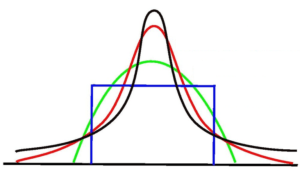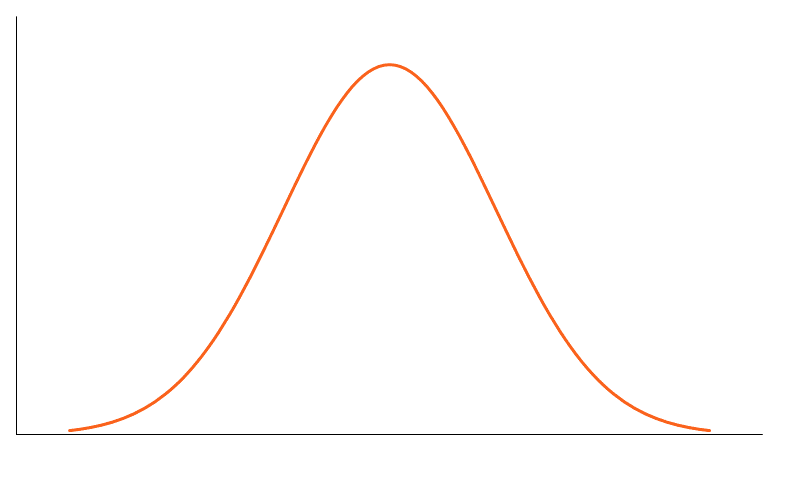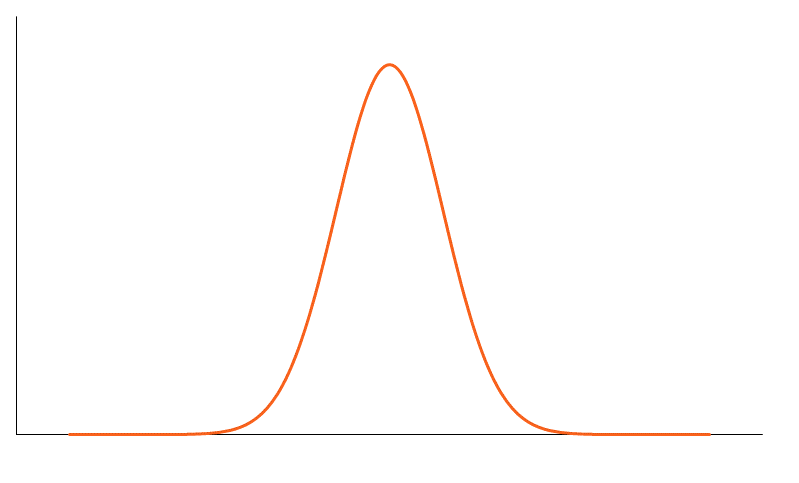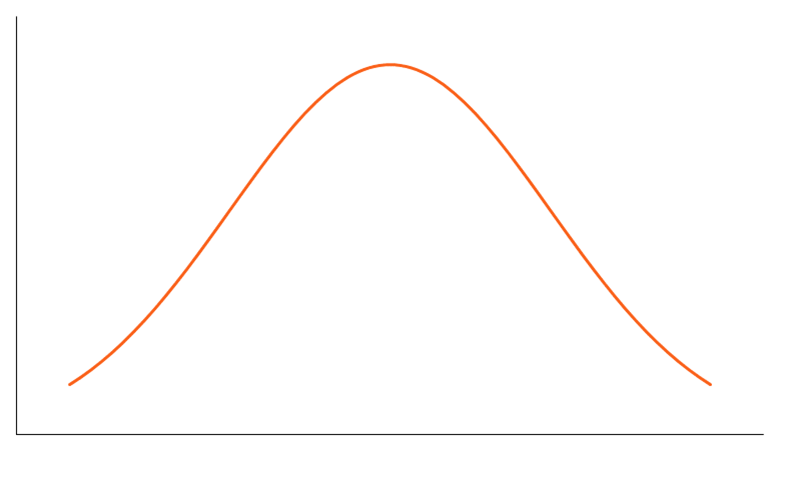# Kurtosis

A statistical measure that defines how heavily the tails of a distribution differ from the tails of a normal distribution

## What is Kurtosis?

Kurtosis is a statistical measure that defines how heavily the tails of a distribution differ from the tails of a normal distribution. In other words, kurtosis identifies whether the tails of a given distribution contain extreme values.Along with skewness, kurtosis is an important descriptive statistic of data distribution. However, the two concepts must not be confused with each other. Skewness essentially measures the symmetry of the distribution, while kurtosis determines the heaviness of the distribution tails.

In finance, kurtosis is used as a measure of financial risk. A large kurtosis is associated with a high level of risk of an investment because it indicates that there are high probabilities of extremely large and extremely small returns. On the other hand, a small kurtosis signals a moderate level of risk because the probabilities of extreme returns are relatively low.

### What is Excess Kurtosis?

An excess kurtosis is a metric that compares the kurtosis of a distribution against the kurtosis of a normal distribution. The kurtosis of a normal distribution equals 3. Therefore, the excess kurtosis is found using the formula below:

Excess Kurtosis = Kurtosis – 3

### Types of Kurtosis

The types of kurtosis are determined by the excess kurtosis of a particular distribution. The excess kurtosis can take positive or negative values, as well as values close to zero.

#### 1. Mesokurtic

Data that follows a mesokurtic distribution shows an excess kurtosis of zero or close to zero. It means that if the data follows a normal distribution, it follows a mesokurtic distribution.#### 2. Leptokurtic

Leptokurtic indicates a positive excess kurtosis. The leptokurtic distribution shows heavy tails on either side, indicating the large outliers. In finance, a leptokurtic distribution shows that the investment returns may be prone to extreme values on either side. Therefore, an investment whose returns follow a leptokurtic distribution is considered to be risky.#### 3. Platykurtic

A platykurtic distribution shows a negative excess kurtosis. The kurtosis reveals a distribution with flat tails. The flat tails indicate the small outliers in a distribution. In the finance context, the platykurtic distribution of the investment returns is desirable for investors because there is a small probability that the investment would experience extreme returns.